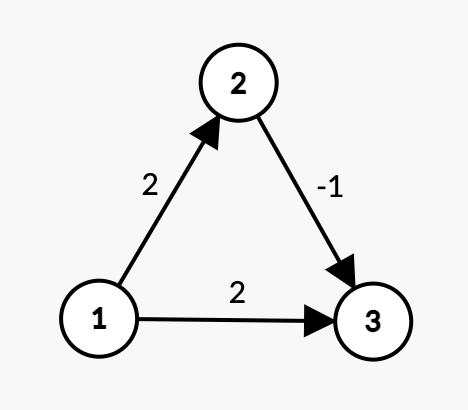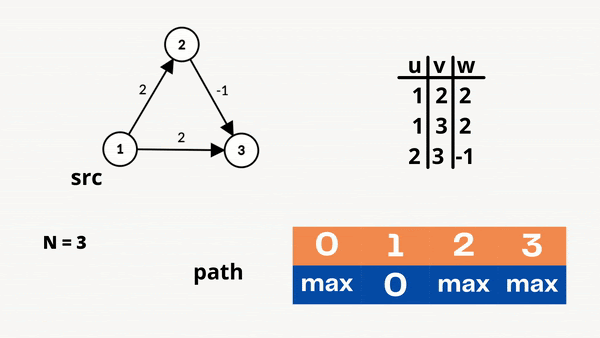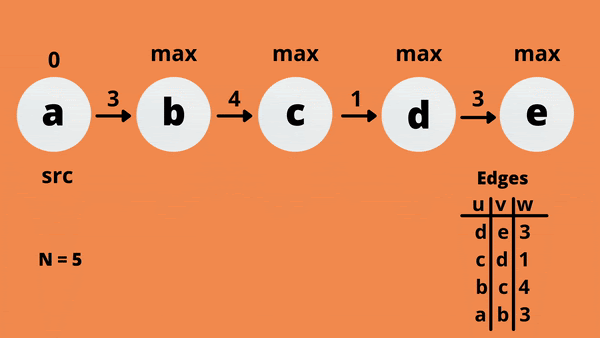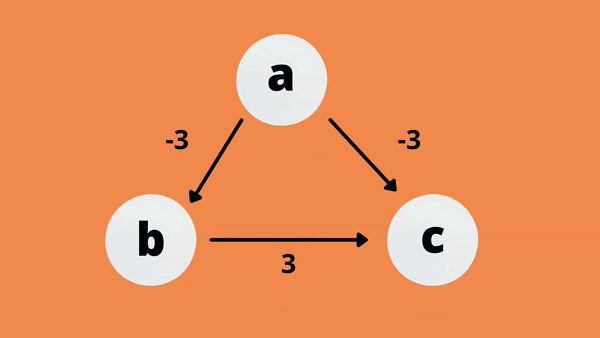# Bellman Ford Algorithm

## Introduction

Consider a case where you are given a weighted graph, i.e., a graph whose edges have weights. You are also given the source and destination points, and now you have to find the shortest path from the source to the destination. You can solve this using the Dijkstra algorithm, but what if the graph contains negative weights? The Dijkstra algorithm will fail in this case.

To solve this problem, an algorithm called the Bellman Ford algorithm would be used. The Bellman Ford Algorithm is used in coding problems like minimum cost maximum flow from a graph, single-source shortest path between two cities, print if there is a negative weight cycle in a directed graph, the path with the smallest product of edges with weight>0, etc.

In this blog, we will cover the Bellman Ford algorithm in detail, along with the code in Java. Since this algorithm is based on graphs, please check out the Introduction to the graph blog to better understand.

## Bellman Ford algorithm

The Bellman-Ford algorithm is similar to the Dijkstra algorithm. These algorithms find the shortest path in a graph from a single source vertex to all the other vertices in a weighted graph. The difference between the Dijkstra and the Bellman-Ford algorithms is that the Bellman-Ford algorithm can be used when the edge weight is negative

The Dijkstra algorithm follows a greedy approach. Once a node is marked as visited, it is not reconsidered even if there is another shortest path. Due to this reason, the Dijkstra algorithm does not work when there is a negative edge weight in the graph.

Now let us understand the Bellman Ford algorithm with the help of an example.

Problem Statement:

A directed weighted graph with 'N' vertices labeled from 1 to 'N' and 'M' edges is given. Each edge connecting two nodes, 'u' and 'v,' has a weight 'w,' denoting the distance between them. The task is to find the shortest path length between the 'source' and 'vertices' given in the graph. Suppose there is no path possible, print 109. The graph may also contain negatively weighted edges.

Consider the directed weighted graph given below and let the source vertex be 1.Directed Weighted Graph

Observations:

• The shortest path length between vertex 1 and vertex 2 is 1->2 with a cost of 2.
• The shortest path length between vertex 1 and vertex 3 is 1->2->3 with a cost of 2 - 1 = 1.

As you might have guessed, we will use the Bellman Ford algorithm to solve the problem.

In the Bellman-Ford algorithm, we overestimate the length of the path from the source vertex to all other vertices. Then the edges are relaxed iteratively by finding new paths that are shorter than the previously overestimated paths. This algorithm uses the concept of dynamic programming and calculates the shortest paths in a bottom-up manner.

Let's look at the algorithm now.

Algorithm:

1. Create an array to store the path length from source to destination.
2. Initialize all distances to maximum value except source.
3. Initialize the distance from source to source as 0.
4. Repeat (N-1) times
1. For every edge from source to destination

if path[v]  > path[u] + weight(uv)

path[v] = path[u] + weight(uv);

Where "u" is the source node, "v" is the destination node, and "weight(uv)" is the edge weight.

If there is no path possible, print 109Working

In this example, the shortest path is obtained in the first iteration. And in the further iteration, there is no change in the path array.

## Bellman Ford Algorithm: Implementation in Java

Let's see how the Bellman Ford algorithm is implemented in Java.

Recommended: Please try to solve the "Bellman-Ford algorithm" on "CODESTUDIO" first before moving on to the solution.

Code:

import java.util.ArrayList;

import java.util.Arrays;

public class Main {

private static void bellmanFord(int n, int m, int src, ArrayList < ArrayList < Integer >> edges) {

// create an array to store the path length from source to i

int[] path = new int[n + 1];

// fill the array with the max value

Arrays.fill(path, Integer.MAX_VALUE);

// distance of source to source is 0

path[src] = 0;

// bellman ford algorithm

for (int i = 1; i < n; i++) {

for (int j = 0; j < m; j++) {

// u node

int u = edges.get(j).get(0);

// v node

int v = edges.get(j).get(1);

// edge weight

int w = edges.get(j).get(2);

// relaxation

if (path[u] != Integer.MAX_VALUE && path[v] > (path[u] + w)) {

path[v] = path[u] + w;

}

}

}

// return the shortest distance from source to all vertices

for (ArrayList < Integer > edge: edges) {

// u node

int u = edge.get(0);

// v node

int v = edge.get(1);

if (u == src) {

path[v] = path[v] == Integer.MAX_VALUE ? 1000000000 : path[v];

System.out.println("The shortest path between " + src + " and " + v + " is: " + path[v]);

}

}

}

// driver code

public static void main(String[] args) {

int n = 3, m = 3, src = 1;

ArrayList < ArrayList < Integer >> edges = new ArrayList < ArrayList < Integer >> ();

ArrayList < Integer > edge1 = new ArrayList < Integer > ();

ArrayList < Integer > edge2 = new ArrayList < Integer > ();

ArrayList < Integer > edge3 = new ArrayList < Integer > ();

bellmanFord(n, m, src, edges);

}

}

Output:

The shortest path between 1 and 2 is: 2

The shortest path between 1 and 3 is: 1

## Bellman Ford algorithm: Complexity Analysis

In the Bellman Ford algorithm, all the edges are relaxed for (N-1) times. So the time complexity is O(M * (N-1)), which can be simplified to O(M * N).

The space complexity of the Bellman Ford algorithm is O(N), as the extra space is required to store the array elements.

Where "M" is the number of edges and "N" is the number of vertices.

## Why (N-1) iterations?

In the Bellman Ford algorithm, there is a chance that the shortest path is obtained before completing (N-1) iterations. However, in the worst case, the shortest path to all the vertices is obtained only at the (N-1)th iteration, which is why we repeat the process of relaxation (N-1) times. Let's see the case where (N-1) iterations are required.As you can see, the shortest path from source "a" to destination "e" is obtained only in the (N-1)th, i.e., the 4th iteration.

## Does it work for negative cycles?

If the total weight of the edges is negative, the weighted graph has a negative cycle. The Bellman Ford algorithm does not work here as there is no shortest path in this case. However, the Bellman Ford algorithm can detect the negative cycle.

Bellman ford relaxes all the edges to find the optimum solution. If there is a negative cycle, the Bellman Ford algorithm will keep ongoing indefinitely. It will always keep finding a more optimized solution, i.e., a more negative value than before. So it becomes necessary to identify the negative cycle.As you can notice, after every cycle, the shortest path keeps on decreasing. To identify the negative cycle, the edges are relaxed again. If the solution is reduced more, there is a negative cycle.

1. Is the Bellman Ford algorithm faster than the Dijkstra algorithm?
Ans:- No, the Dijkstra algorithm is faster than the Bellman Ford algorithm. The time complexity of the Dijkstra algorithm is O(M * log N), and the time complexity of the Bellman Ford algorithm is O(M * N).
Where "M" is the number of edges and "N" is the number of vertices.

2. In the Bellman Ford algorithm, why is the source vertex path set to 0 and other vertices path to the maximum value?
Ans:- The source vertex path is set to 0 as the distance between source to source is 0. The path of other vertices is set to maximum because the distance to each node initially is unknown, so we assign the highest value possible.

3. How is the Dijkstra algorithm different when compared to the Bellman Ford algorithm?
Ans:- The difference between the Dijkstra algorithm and  Bellman Ford algorithm are:-

• The Dijkstra algorithm uses the greedy approach, whereas the Bellman Ford algorithm uses dynamic programming.

• In the Dijkstra algorithm, the minimum value of all vertices is found out, while in the Bellman-Ford algorithm, edges are considered one by one.

## Key Takeaways

This blog covers the famous graph algorithm, the Bellman Ford algorithm, along with the implementation in Java. Frequently asked questions based on the Bellman Ford algorithm have also been added to this blog.

Also, check out the other algorithms based on graphs like Floyd Warshall Algorithm, Prim's Algorithm, Kruskal's Algorithm, Dijkstra's Algorithm, etc. Now that you know the various algorithms based on graphs, try out some questions on our CodeStudio Platform!

Don't stop here. Check out our Data Structures and Algorithm - guided path to learning Data Structures and Algorithms from scratch. We hope you found this blog useful. Feel free to let us know your thoughts in the comments section.